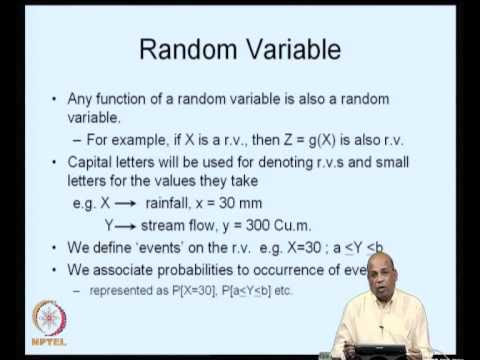### Stochastic HydrologyThe objective of this course is to introduce the concepts of probability theory and stochastic processes with applications in hydrologic analysis and design.
Modeling of hydrologic time series with specific techniques for data generation and hydrologic forecasting will be dealt with.
Case study applications will be discussed.

 Topic No. of Hours Introduction to Random Variables (RVs). 01 Probability Distributions - One dimensional RVs. 02 Higher Dimensional RVs - Joint Distribution. 02 Conditional Distribution; Independence. 03 Properties of Random Variables. 02 Parameter Estimation. 02 Commonly used Distributions in Hydrology. 05 Hydrologic Data Generation. 04 Introduction to Time Series - stationarity; ergodicity. 02 Purely stochastic Models; Markov Processes. 05 Spectral Density; Analysis in the Frequency Domain. 04 Auto Correlation and Partial Auto Correlation. 02 Auto Regressive Moving Average Models (Box - Jenkins models - model identification; Parameter estimation ; calibration and validation; Simulation of hydrologic time series ; Applications to Hydrologic Forecasting - case studies). 06 Total 40